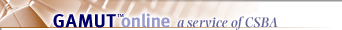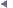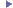Legal Resources | Education Code |  EC  54023

Economic Impact AidPreviousNextFor each fiscal year, the economic impact aid-eligible pupil count shall be calculated for each school district as follows:

(a) Determine the count of economically disadvantaged pupils, as defined in Section 54026.

(b) Determine the count of English learners, as defined in subdivision (b) of Section 54026.

(c) Calculate an economic impact aid weighted pupil concentration factor:

(1) Add the pupil counts determined in subdivisions (a) and (b).

(2) Divide the fall CBEDS enrollment for the school district for the prior school year by two.

(3) Subtract from the sum calculated in paragraph (1) the quotient calculated in paragraph (2).

(4) If the result of the calculation in paragraph (3) is greater than zero, multiply that difference by 0.5. If the result is less than zero, it shall be deemed to be zero.

(d) The economic impact aid-eligible pupil count for each school district shall equal the sum of the pupil counts determined in subdivisions (a) and (b), and the weighted pupil concentration factor determined in subdivision (c).

(e) In calculating the economic impact aid-eligible pupil count for a new charter school in its first year of operation, the department shall use CBEDS enrollment counts and counts of English learners reported in the current year instead of the prior year.

(Amended by Stats. 2007, Ch. 730, Sec. 35.)

Reference:

Education Code 54026